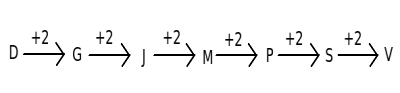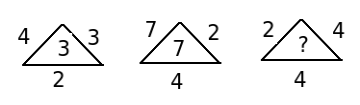# Model Aptitude questions and answers for placement - Set 16

1)   In given question, various terms of an alphabet series is given with one term missing as shown by (?). Choose the missing term out of the given alternatives.

D,  G,  J,  M,  ?,  S,  V

a. N
b. P
c. K
d. O
e. R
 Answer  Explanation ANSWER: P Explanation: Given:D, G, J, M, ?, S, VRefer : A B C D E F G H I J K L M N O P Q R S T U V W X Y Z We have,2)   If 1.5 a = 0.03 b, then the value of (b – a)/ (b + a) is

a. 490/51
b. 49/51
c. 4.9/51
d. None of these
 Answer  Explanation ANSWER: 49/51 Explanation: a/b = 0.03/1.5 = 3/150 = 1/50 (b – a)/(b + a) = (1 – a/b)/((1 + a/b)= (1 – 1/50)/ (1 + 1/50) = 49/51

3)   A grocer has a sale of Rs. 5420, Rs. 5660, Rs. 6200, Rs. 6350 and Rs. 6500 for 5  consecutive months. Find the sale he should have in the sixth month, so that he gets an average sale of Rs. 6000?

a. Rs. 5870
b. Rs. 5991
c. Rs. 6020
d. Rs. 6850
 Answer  Explanation ANSWER: Rs. 5870 Explanation: Total sale for 5 months = Rs. (5420 + 5660 + 6200 + 6350 + 6500) = Rs. 30,130Therefore, required sale = Rs. [(6000 * 6) – 30,130] = Rs. (36000 – 30,130) = Rs. 5870

4)   If “GREEN” is given number code as 49, what is the difference between the number codes of “VIOLET” and “PINK” ?

a. 55
b. 45
c. 33
d. 60
e. 40
 Answer  Explanation ANSWER: 33 Explanation: Case – Codes : A - 1, B - 2, C - 3, D - 4, E - 5, F - 6, G - 7, H - 8, I - 9, J - 10, K - 11, L - 12, M - 13, N - 14, O - 15, P - 16, Q - 17, R - 18, S - 19, T - 20, U - 21, V - 22, W - 23, X - 24, Y - 25, Z - 26GREEN = 7 + 18 + 5 + 5 + 14 = 49Thus we get,VIOLET = 22 +9 +15+12 + 5 + 20 = 83PINK = 16 + 9 + 14 + 11 = 50Thus, difference is 83 – 50 = 33.

5)   Find Nikhil's present age, if

I. Five years ago, Nikhil's age was double that of his daughter's age at that time.
II. Present ages of Nikhil and his daughter are in the ratio of 11:6 respectively
III. Five years hence, the respective ratio of Nikhil's age and his daughter age will become 12:7

a. Only I and II
b. Only II and III
c. Only I and III
d. Any two of the three
 Answer  Explanation ANSWER: Any two of the three Explanation: 1) Present ages of Nikhil and his daughter will be 11x and 6x years respectively.2) 5 years ago, Nikhil's age = 2 * His daughter's age3) 5 years hence, Nikhil's age / daughter's age = 12/7 Clearly, any two of the above will give Nikhil's present age Therefore, correct answer is (d).

6)   Sam, Suresh and Sanket run a race of 1400 meters. Sam runs twice as fast as Suresh while Suresh runs thrice as fast as Sanket. If Sanket finishes the race by clocking 15 minutes, how far was Suresh from the finish line when Sam must have finished the race?

a. 450 m
b. 680 m
c. 700 m
d. 900 m
e. 1000 m
 Answer  Explanation ANSWER: 700 m Explanation: When Sanket finishes the race in 15 minutes, Suresh & Sam would finish the same race in 5 minutes and 2.5 minutes respectively. Thus, when Sam reaches the ”finish” mark, Suresh must be “half-way” through i.e. 700 meters behind the “finish” line when “Sam” must have finished the race.

7)   How many 7's are present in the following sequence of numbers which are both preceded and followed by perfect square numbers ?

8  6  3  7  9  7  4  3  6  9  7  2  1  7  4  9  5  2  5  4  7  9  5  2  9  7  1  4  7  1

a. 4
b. 2
c. 5
d. 3
e. None of these
 Answer  Explanation ANSWER: 5 Explanation: 8 6 3 7 9 7 4 3 6 9 7 2 1 7 4 9 5 2 5 4 7 9 5 2 9 7 1 4 7 1

8)   What approximate value should come in place of (x) in the following question?
139.25 + 223.94 / 4.002 = x

a. 250
b. 203
c. 200
d. 195
 Answer  Explanation ANSWER: 195 Explanation: Given: 139.25 + 223.94 / 4.002 = x Approximate 139.25 as 140, 223.94 as 224, 4.002 as 4,we get, x = 140 + 224 / 4 = 140 + 56 = 196 =>195

9)   A box contains 4 black, 3 red and 6 green marbles. 2 marbles are drawn from the box at random. What is the probability that both the marbles are of the same color?

a. 12/74
c. 13/78
d. None of these
 Answer  Explanation ANSWER: 24/78 Explanation: Total marbles in a box = 4 black + 3 red + 6 green marbles = 13 marbles2 marbles are drawn from 13 marbles at random. Therefore, n(S) = 13C2 = 78 waysLet A be the event that 2 marbles drawn at random are of the same color. Number of cases favorable to the event A isn(A) = 4C2 + 3C2 + 6C2 = 6 +3 + 15 = 24Therefore, by definition of probability of event A,P(A) = n(A)/n(S) = 24/78

10)   In an examination, the percentage of students qualified to the students appeared from school 'P' is 70%. In school 'Q', the number of students appeared is 30% more than the students appeared from school 'P' and the number of students qualified from school 'Q' is 50% more than the students qualified from school 'P'. What is the % of students qualified to the number of students appeared from school 'Q' ?

a. 80.76%
b. 75.5%
c. 60.50%
d. 43 %
e. None of these
 Answer  Explanation ANSWER: 80.76% Explanation: Number of students appeared from school 'P' = 100, sayNumber of students qualified from school 'P' = 70and Number of students appeared from school 'Q' = 130Number of students qualified from school 'Q' = 50% more than those qualified from school 'P'.= 70 + 35 = 105 % of students qualified to the number of students appeared from school B = 105 / 130 * 100= 80.76%

11)   Kiran started a business investing Rs. 20,000. After 4 months, Sanket joined with a capital of Rs. 12000. At the end of the year, they earn a profit of Rs. 14,000. Find what will be the share of Sanket in the profit?

a. Rs. 2880
b. Rs. 3000
c. Rs. 4000
d. Rs. 3700
e. Rs. 3560
 Answer  Explanation ANSWER: Rs. 4000 Explanation: Kiran : Sanket = (20000 * 12) : (12000 * 8) = 5 : 2 Sanket's share = Rs. 14,000 * (2/7) = Rs. 4000

12)   Find the missing numbera. 5
b. 8
c. 4
d. 2
e. None of these
 Answer  Explanation ANSWER: 4 Explanation: We have :4 * 3 * 2 / 8 = 24/8 = 37 * 2 * 4 / 8 = 56/8 = 7Thus, missing number = 2 * 4 * 4/8 = 32/8 = 4